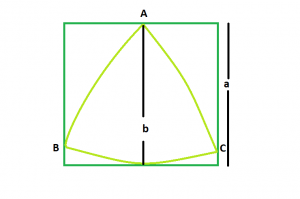GFG App
Open AppBrowser
Continue

# Biggest Reuleaux Triangle within A Square

Given an integer a which is the side of a square, the task is to find the biggest Reuleaux Triangle that can be inscribed within it.Examples:

Input: a = 6
Output: 25.3717

Input: a = 8
Output: 45.1053

Approach: We know that the Area of Reuleaux Triangle is 0.70477 * b2 where b is the distance between the parallel lines supporting the Reuleaux Triangle.
From the figure, it is clear that distance between parallel lines supporting the Reuleaux Triangle = Side of the square i.e. a
So, Area of the Reuleaux Triangle, A = 0.70477 * a2

Below is the implementation of the above approach:

## C++

 `// C++ Program to find the area` `// of the biggest Reuleaux triangle` `// that can be inscribed within a square` `#include ` `using` `namespace` `std;`   `// Function to find the Area` `// of the Reuleaux triangle` `float` `ReuleauxArea(``float` `a)` `{`   `    ``// Side cannot be negative` `    ``if` `(a < 0)` `        ``return` `-1;`   `    ``// Area of the Reuleaux triangle` `    ``float` `A = 0.70477 * ``pow``(a, 2);` `    ``return` `A;` `}`   `// Driver code` `int` `main()` `{` `    ``float` `a = 6;` `    ``cout << ReuleauxArea(a) << endl;` `    ``return` `0;` `}`

## Java

 `// Java Program to find the area` `// of the biggest Reuleaux triangle` `// that can be inscribed within a square` `import` `java.lang.Math;` `class` `cfg ` `{` `// Function to find the Area` `// of the Reuleaux triangle` ` ``static` `double` `ReuleauxArea(``double` `a)` `{`   `    ``// Side cannot be negative` `    ``if` `(a < ``0``)` `        ``return` `-``1``;`   `    ``// Area of the Reuleaux triangle` `    ``double` `A = ``0.70477` `* Math.pow(a, ``2``);` `    ``return` `A;` `}`   `// Driver code` `public` `static` `void` `main(String[] args)` `{` `    ``double` `a= ``6``;` `    ``System.out.println(ReuleauxArea(a) );` `    `  `}` `}``//This code is contributed by Mukul Singh. `

## Python3

 `# Python3 Program to find the area ` `# of the biggest Reuleaux triangle ` `# that can be inscribed within a square `   `# Function to find the Area ` `# of the Reuleaux triangle ` `def` `ReuleauxArea(a) :`   `    ``# Side cannot be negative ` `    ``if` `(a < ``0``) :` `        ``return` `-``1`   `    ``# Area of the Reuleaux triangle ` `    ``A ``=` `0.70477` `*` `pow``(a, ``2``); ` `    ``return` `A`   `# Driver code ` `if` `__name__ ``=``=` `"__main__"` `:`   `    ``a ``=` `6` `    ``print``(ReuleauxArea(a)) `   `# This code is contributed by Ryuga`

## C#

 `// C# program to find area of the ` `//biggest  Reuleaux triangle that can be inscribed` `//within a square` `using` `System; ` `  `  `class` `GFG { ` `  `  `    ``// Function to find the area ` `    ``// of the reuleaux triangle ` `    ``static` `double` `reuleauxArea(``double` `a) ` `    ``{ ` `  `  `     ``//Side cannot be negative` `     ``if` `(a<0)` `        ``return` `-1;` `        `  `        ``// Area of the reuleaux triangle ` `        ``double` `A=0.70477*Math.Pow(a,2);` `        ``return` `A;` `    ``} ` `  `  `    ``// Driver code ` `    ``static` `public` `void` `Main() ` `    ``{ ` `        ``double` `a= 6; ` `        ``Console.WriteLine(reuleauxArea( a)); ` `    ``} ` `} ` `//This code is contributed by Mohit kumar 29`

## PHP

 ``

## Javascript

 ``

Output:

`25.3717`

Time Complexity: O(1)

Auxiliary Space: O(1)

My Personal Notes arrow_drop_up